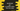# 3 ways to get a random value from an array in JavaScript## How to get a random value from an array in JavaScript:

You might need to get a random value from an array in JavaScript. Arrays are used to store data in continuous memory locations. The index can be used to access an element in an array. It starts from 0 and ends at length of the array - 1, i.e. the index of the first element is 0, the index of the second element is 1, third element is 2 etc.

If we want to pick a random value from an array, we need to create a random index. Once we get the index, it is easy to get the value at that index.

The Math.random() is an inbuilt function to create a random number in JavaScript. This function can be used to create a random value at any given range. We can use this function with other different functions to pick a random value from a JavaScript array.

Let me show you 3 different ways to do that.

### Method 1: By using Math.random():

Let’s learn how Math.random works. This function is defined as like below:

``Math.random()``

It doesn’t take any parameters. It returns a floating-point, pseudo-random number between 0 and 1. 0 is inclusive and 1 is exclusive i.e. the number will be always in the range of 0 to less than 1.

But, if we want to pick a random value in an array, we need a number between 0 to length of the array - 1. For that, we can use the below formula:

``Math.floor(Math.random() * arrayLength)``

Math.floor returns the largest integer less than or equal to a given number.

To get the length of an array, we can read the length property. array.length returns the length of an array.

Let’s write down the program:

``````const getRandom = (arr) => {
return arr[Math.floor(Math.random() * arr.length)];
};

let arr = [
"🐟",
"🐳",
"🐋",
"🦈",
"🍇",
"🍓",
"🫐",
"🍒",
"🍑",
"🍫",
"🍬",
"🍭",
];

console.log(getRandom(arr));``````

In this program,

• getRandom method is used to get a random value from the array.
• It uses Math.floor and Math.random to get a random index in the array arr.
• arr[] returns the random item in the array.

If you run this program, it will print a random smiley each time you run it.

### Method 2: By using ~~ operator:

The ~~ operator can be used to convert a value to integer. We can use it instead of Math.floor. It is faster than Math.floor. Let me change the above program to use it instead of Math.floor:

``````const getRandom = (arr) => {
return arr[~~(Math.random() * arr.length)];
};

let arr = [
"🐟",
"🐳",
"🐋",
"🦈",
"🍇",
"🍓",
"🫐",
"🍒",
"🍑",
"🍫",
"🍬",
"🍭",
];

console.log(getRandom(arr));``````

It will give a similar output. It will print a random value in arr each time you run this program.

### Method 3: Using lodash:

If you are using lodash in your project, you can use it. It provides a method called _.sample() that returns a random element from a collection. We can use this method with an array.

For example,

``````import _ from 'lodash';

let arr = [
"🐟",
"🐳",
"🐋",
"🦈",
"🍇",
"🍓",
"🫐",
"🍒",
"🍑",
"🍫",
"🍬",
"🍭",
];

console.log(_.sample(arr));``````

It will print a random value from the array arr each time you run it.

### Conclusion:

In this post, we learned three ways to get a random element in an array in JavaScript. You can use any of these methods. All of these will give a random array element.